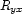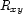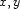dom method

This method translates a dominance literal dom(X R Y) into the corresponding problem-specific constraint (as described in Section 4.3.2). Remember that sinceis the inverse of, we only represent one of them: we representwhenare encoded by I,J and I>J.

meth dom(X R Y)
{self var2node(X _)}
{self var2node(Y _)}
I = @var2int.X
J = @var2int.Y
in
if I==then 1::{Encode R}
elseif I>then
@choices.(I*1000+J)::{Encode R}
else
@choices.(J*1000+I)::{Encode {Inverse R}}
end
end

Here is how to inverse and encode the symbolic representation of a dominance specification.

<<
fun {Encode R}
case R
of eq    then 1
[] above then 2
[] below then 3
[] side  then 4
[] _|_   then {Map R Encode}
end
end
fun {Inverse R}
case R
of eq    then eq
[] above then below
[] below then above
[] side  then side
[] _|_   then {Map R Inverse}
end
end

Denys Duchier
Version 1.2.0 (20010221)